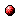# Distributed model predictive control for rescheduling of railway traffic

Reference:
B. Kersbergen, T. van den Boom, and B. De Schutter, "Distributed model predictive control for rescheduling of railway traffic," Proceedings of the 17th International IEEE Conference on Intelligent Transportation Systems (ITSC 2014), Qingdao, China, pp. 2732-2737, Oct. 2014.

Abstract:
In this paper we introduce two distributed model predictive control (DMPC) methods for the rescheduling of railway traffic. In each step of the DMPC approach dispatching actions are determined that reduce the amount of delay in the network as much as possible by solving a mixed integer linear programming (MILP) problem. The constraints of the MILP are based on a model of the railway traffic and network and the possible dispatching actions. In the first method each subproblem consists of the complete constraint matrix and the solver tries to minimize the centralized cost function, but can only change a limited number of binary variables (which correspond to the dispatching actions). By limiting the number of binary variables each subproblem is easier to solve than the centralized problem. For the second method each subproblem consists of only a part of the problem and the solver minimizes a local cost function, and it can only change the binary variables for that part of the problem. This reduces the complexity of the subproblems even further, but the solver can not determine the effects of the binary variables on the solution quality of the other subproblems. Both methods significantly reduce the time needed to determine the dispatching actions. The average time needed to compute the solution is 11.56 times shorter when using method 1 and 39.11 times shorter when using method 2. The solution found is on average only 0.63% less optimal for method 1 and 1.27% less optimal for method 2.Online version of the paperCorresponding technical report: pdf file (341 KB)
Note: More information on the pdf file format mentioned above can be found here.

Bibtex entry:

@inproceedings{Kervan:14-036,
author={B. Kersbergen and T. van den Boom and B. {D}e Schutter},
title={Distributed model predictive control for rescheduling of railway traffic},
booktitle={Proceedings of the 17th International IEEE Conference on Intelligent Transportation Systems (ITSC 2014)},